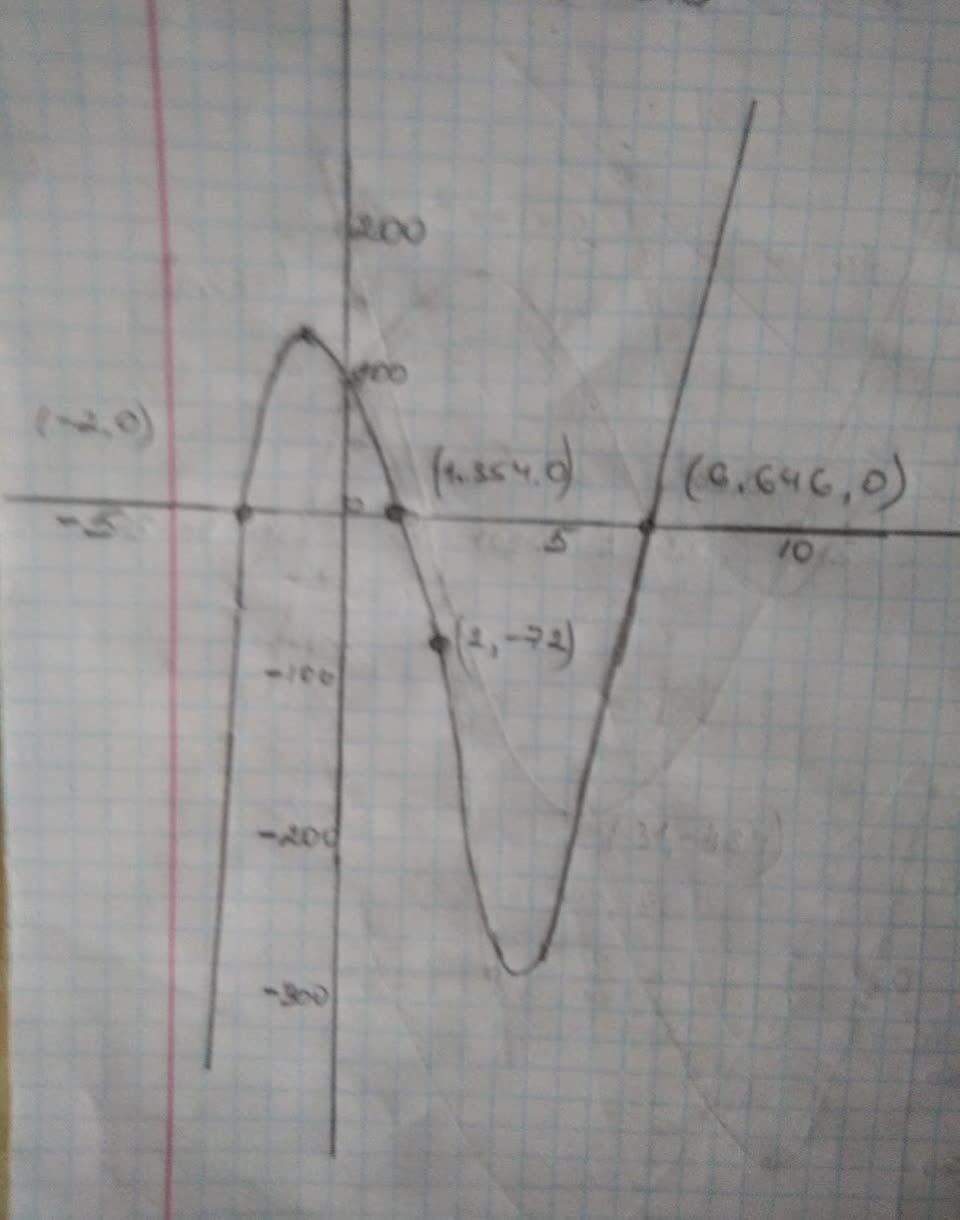Judith McQueen

2021-12-07

A family of cubic functions have zeros of -2 and $4±\sqrt{7}$.
4) Write the equation ofthe family of cubic Functions in factored form. The equation may contain a ‘non-factorable quadratic but should contain no radicals. Show your work,
b) Write the equation for the member which goes through the point (2, -72). Show vour work,
c) Describe the end behaviour for the member in b).Ana Robertson

Step 1
Family of cubic functions that have the roots a,b and c is $y=D\left(x-a\right)\left(x-b\right)\left(x-c\right)$, here D is a constant.
Given roots are: .
(a) $y=D\left(x-\left(-2\right)\right)\left(x-\left(4+\sqrt{7}\right)\right)\left(x-\left(4-\sqrt{7}\right)\right)$
$=D\left(x+2\right)\left(\left(x-4\right)-\sqrt{7}\right)\left(\left(x-4\right)+\sqrt{7}\right)$
$=D\left(x+2\right)\left({\left(x-4\right)}^{2}-7\right)\left(A-B\right)\left(A+B\right)$
$={A}^{2}-{B}^{2}$
$=D\left(x+2\right)\left({x}^{2}+16-8x-7\right){\left(A-B\right)}^{2}={A}^{2}+{B}^{2}-2AB$
$=D\left(x+2\right)\left({x}^{2}-8x+9\right)$
Step 2
(b) Substitute and solve for D.
$-712=D\left(2+2\right)\left({2}^{2}-8\left(2\right)+9\right)$
$6=D$
For cubic equation which goes through point (2, -72) substitute
we get, $y=6\left(x+2\right)\left({x}^{2}-8x+9\right)$
The graph $y=6\left(x+2\right)\left({x}^{2}-8x+9\right)$ shows the cubic equation passing through the point (2, -72) which have
the three roots .Do you have a similar question?